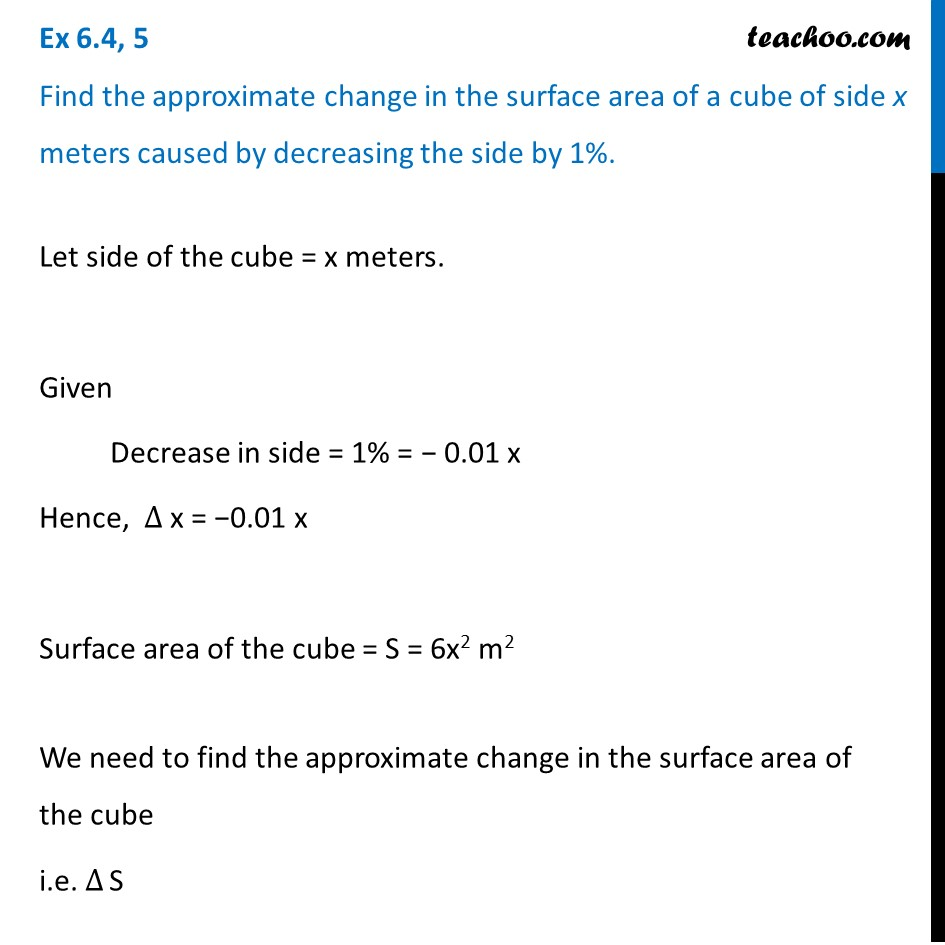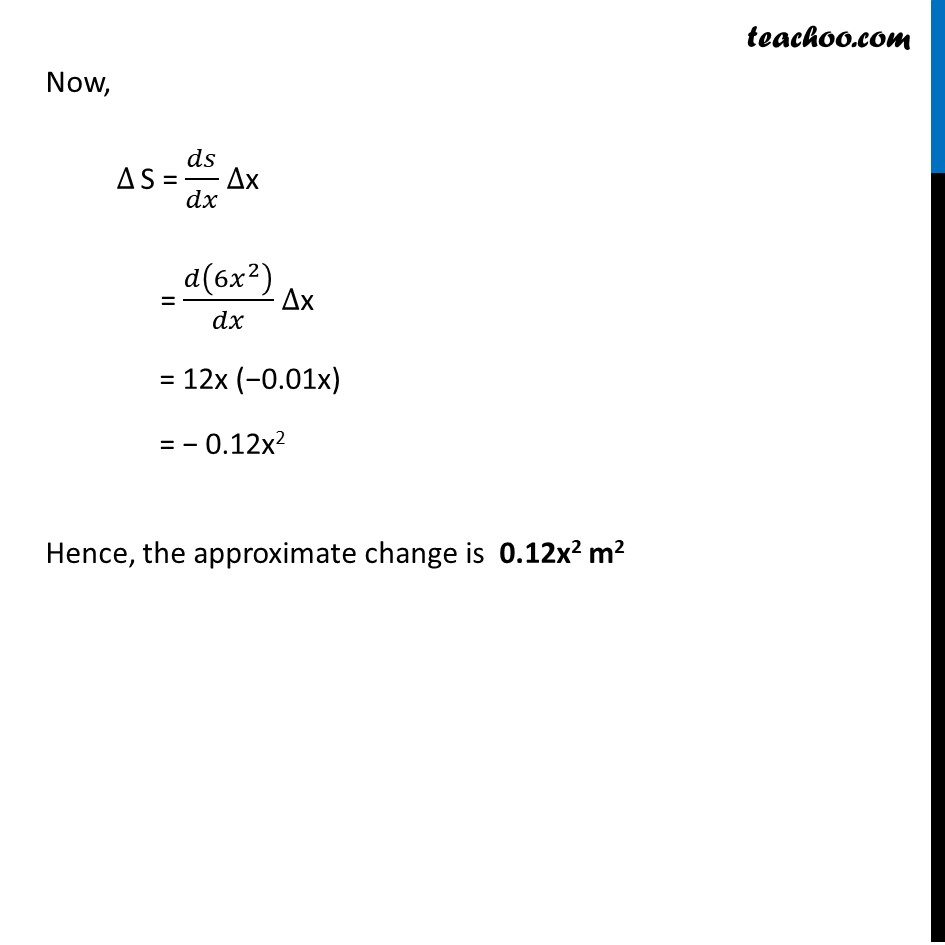Approximations (using Differentiation)

Chapter 6 Class 12 Application of Derivatives
Serial order wiseLearn in your speed, with individual attention - Teachoo Maths 1-on-1 Class

### Transcript

Question 5 Find the approximate change in the surface area of a cube of side x meters caused by decreasing the side by 1%.Let side of the cube = x meters. Given Decrease in side = 1% = − 0.01 x Hence, ∆ x = −0.01 x Surface area of the cube = S = 6x2 m2 We need to find the approximate change in the surface area of the cube i.e. ∆ S Now, ∆ S = 𝑑𝑠/𝑑𝑥 ∆x = 𝑑(6𝑥^2 )/𝑑𝑥 ∆x = 12x (−0.01x) = − 0.12x2 Hence, the approximate change is 0.12x2 m2